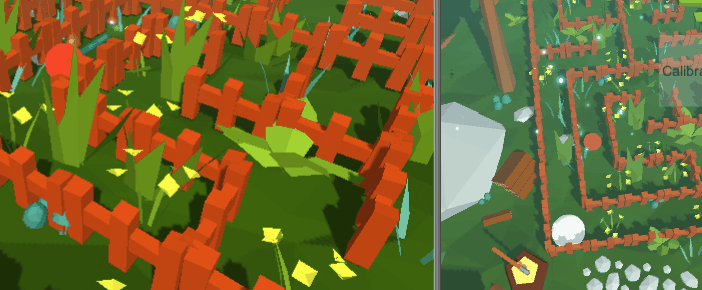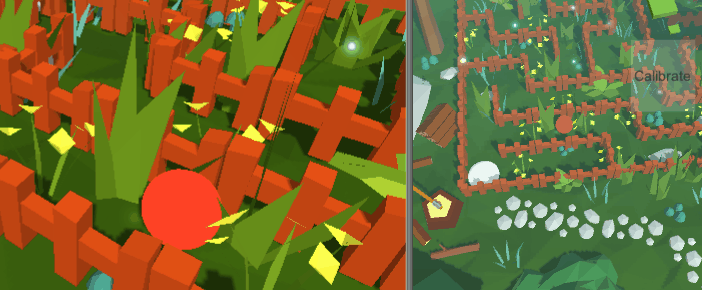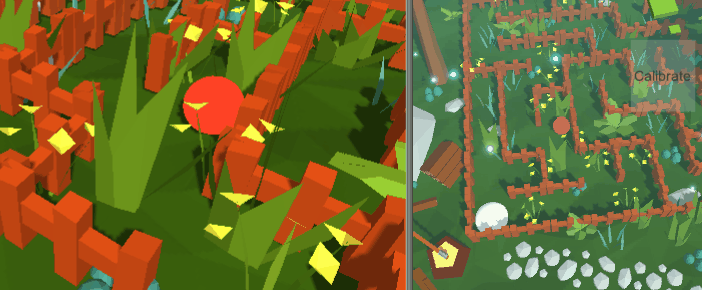Here’s what we’ll be making:I recently started playing Pokemon: Let’s Go Eevee for the Nintendo Switch, and I’ve been having a great time. There’s this neat effect in Let’s Go Eevee where the grass, trees, and flowers squish when the player walks over them. There’s a clip of it here. Inspired by this, I decided to implement a similar effect in my own game!

If you’re unfamiliar with writing shaders, you might want to check out Alan Zucconi’s introduction to writing shaders in Unity. There’s a ton of resources on his site, and I’ve been reading them as I’ve been learning.

Now on to the effect! There are a bunch of different ways to approach squishy grass shaders. I’ll go through a few of them that I tried and their pros/cons.

### Single Position Vector (Using Vertex Distance)

The simplest solution is to pass the player’s position to the shader as a uniform variable, and displace the vertices downward if they are within a radius of that position in the vertex shader. We can use the distance of the player to the vertex in our calculations. The C# code for this is below.

``````Shader.SetGlobalVector("_PlayerWorldPos", transform.position);
``````

And here is the vertex shader code where the (vertex displacement occurs).

``````uniform float _DisplacementStrength;
uniform float4 _PlayerWorldPos;
void vert(inout appdata_full v) {
// check if this vertice's world position is within a certain distance of the player
float d = distance(mul(unity_ObjectToWorld, v.vertex).xz, _PlayerWorldPos.xz);
// if it is, displace this vertex down, in relation to its distance to the player
v.vertex.y -= (1. - d / _Radius) * _DisplacementStrength * .5 * saturate(v.vertex.y);
// but don't go below the ground
v.vertex.y = max(0.05, v.vertex.y);
}
}
``````Using one position vector like this might be good for a distortion effect, but not for grass. Object meshes appear broken when half of their vertices are near the player and the other half are unaffected. To make a better squishy-grass behaviour, we can check if the player is within a radius of the vertex using the vertices object position instead of its world position.

### Single Position Vector (Using Object Distance)

To transform the player’s world position into object space, we will use the unity_WorldToObject matrix that Unity provides as a global variable. This will give us the player’s distance from the center of the object. Using this distance, the vertices of that object are displaced downwards in the vertex shader. A _SquishThreshold variable is added here as well, such that vertices which are originally above the threshold will never be displaced lower than it when squished.

The C# code stays the same as when using the vertex distance, since we are still using the player position vector, however the vertex shader code is updated as seen below. Note the use of the unity_WorldToObject matrix here.

``````uniform float _SquishThreshold;
uniform float _DisplacementStrength;
uniform float4 _PlayerWorldPos;
float getPlayerObjectDistance() {
float2 pPos = mul(unity_WorldToObject, _PlayerWorldPos).xz;
return length(pPos);
}
void vert(inout appdata_full v) {
// check if this object's center is within a certain distance of the player
float d = getPlayerObjectDistance();
float originalVertY = v.vertex.y;
float intensity = 1. - abs(d / (float)_Radius);
v.vertex.y -= intensity * _DisplacementStrength * .5 * saturate(v.vertex.y);
if (originalVertY > v.vertex.y) {
v.vertex.y = max(_SquishThreshold, v.vertex.y);
}
return;
}
else return;
}
``````This effect works well and is performant, but the grass stops being squishy once the player leaves the radius of the object. We can get around this limitation in the following solution using a vector array.

### Multi-Position Vector Array (Using Object Distance)

The multi-position vector array technique involves passing the player’s previous positions over the past several frames (as a vector array) to the vertex shader, and then displacing the vertices based on the number of previous player positions were close to the object. I found that this technique produced the most similar to Pokemon Eevee’s squishy grass, but is the most expensive, since it requires a vector array instead of a single vector.

In addition, the intensity with which this effect is applied can be changed from linear to cubic to get a fast-in, slow-out effect. The GLSL Grapher website helped to arrive at a good function for this.

Here is the new C# code for passing in a vector array. The reason why the PWShiftRight() and PWPush() functions are implemented here is because the shader wasn’t accepting the array when I was using the System.Collections Queue class. I was getting an error saying that the new array size of 20 did not match the previous array size of 1. The solution to this for my version of Unity (2018.2.10f) is to use a fixed-size, global array in my C# program, and restart the Unity editor whenever I change this fixed array size. For further reading on passing vector arrays to shaders in Unity, including its limitations, see Alan Zucconi’s blog post on the topic (I found this post to be still relevant, even though it is about Unity 5.4).

``````public class Player : Monobehaviour {
Vector4[] playerWorldPosArray = new Vector4;
void Awake () {
// initialize the global array of player world positions
for (var i = 0; i < playerWorldPosArray.Length; i++) {
playerWorldPosArray[i] = new Vector4(
transform.position.x,
transform.position.y,
transform.position.z,
1);
}
StartCoroutine(UpdateWorldPos());
}
IEnumerator UpdateWorldPos() {
while (true) {
// get the most recent player world position
var wp = new Vector4(
transform.position.x,
transform.position.y,
transform.position.z,
1);
// dequeue the last position from the global position array
PWShiftRight();
// enqueue the new position on to the global position array
PWPush(wp);
// set our position array as a global shader variable -
yield return new WaitForEndOfFrame();
}
}
// See the reasoning above for why queue operations are implemented here
// PWShiftRight() dequeues from the end of the global position array
void PWShiftRight() {
for(var i=playerWorldPosArray.Length-1; i>=1; i--) {
playerWorldPosArray[i] = playerWorldPosArray[i - 1];
}
}
// PWPush(v) enqueues v on to the start of the global position array
void PWPush(Vector4 v) { playerWorldPosArray = v; }
#if UNITY_EDITOR
private void OnDrawGizmos()
{
// This draws the player position array in the Unity editor
Gizmos.color = Color.cyan - (Color.black * .5f);
for(var i=0; i<playerWorldPosArray.Length; i++) {
var pos = new Vector3(playerWorldPosArray[i].x,
playerWorldPosArray[i].y,
playerWorldPosArray[i].z);
Gizmos.DrawSphere(pos, .05f);
}
}
#endif
}
``````

``````#define P_LENGTH 20
uniform float4 _PlayerWorldPos[P_LENGTH];
uniform float _DisplacementStrength;
uniform float _SquishThreshold;
float getPlayerObjectDistance(int pIndex) {
float2 pPos = mul(unity_WorldToObject, _PlayerWorldPos[pIndex]).xz;
return length(pPos);
}
void vert(inout appdata_full v) {
// check if this object is within a certain distance of the player
float d = getPlayerObjectDistance(0);
if (d > (_Radius * P_LENGTH * .3)) {
// ignore this vertex if the player is too far away
return;
}
// check how many player positions are within range of this object
int numPFramesContained = 0;
for (int i = 1; i < P_LENGTH; i++) {
}
float originalVertY = v.vertex.y;
// displace this vertex down a certain intensity based on how long the player has been near this object
float intensity = (numPFramesContained / (float)P_LENGTH);
// we transform intensity here with a cubic function for the fast-in, slow-out effect
intensity -= 1.;
intensity = 1. + (intensity*intensity*intensity);
v.vertex.y -= intensity * _DisplacementStrength * .5 * saturate(v.vertex.y);
if (originalVertY > v.vertex.y) {
v.vertex.y = max(_SquishThreshold, v.vertex.y);
}
}
``````This effect appears the most similar to Pokemon’s out of the three presented here, but if performance is an issue, the second solution may be substituded for a similar result. The P_LENGTH variable can also be reduced to improve performance. The blue dots on the left of the gif are the positions of the player from the previous 20 frames. You can see the effect they have on the grass even once the player has left the object’s radius. Mission Complete 😄!

Thank you for stopping by, and I hope you enjoyed my bouncy grass shaders! Stay tuned for future blog posts and games, including this one, by following Restaurant On Mars Studios’ Facebook Page.

Thank you!

Nicholas Bucher,

CEO, Restaurant On Mars Studios